On the Topology of Celtic Knot Designs

Gwen Fisher

Mathematics Department

California Polytechnic State University

San Luis Obispo, CA 93407

Blake Mellor

Mathematics Department

Loyola Marymount University

Los Angeles, CA 90045-2659

Abstract

 We derive formulas for counting the number of strands in a variety of knotwork designs inspired by traditional Celtic designs, including rectangular panels, circular borders, rectangular borders, and half frames. We include graphic examples for each of these types of designs.

1. Introduction

There is a long tradition of abstract geometric designs in the art of the Celtic peoples of ancient Britain, Scotland and Ireland, including spirals, key patterns and, in the Christian era, knots and interlacings . Complex knotwork patterns were used profusely in the Celtic illuminated manuscripts, such as in the Books of Durrow (early 5th to early 6th century), Kells (middle 6th to early 8th century), Lindesfarne (late 7th century), and Grimbald of St. Bertin (early 11th century). In these manuscripts, interlacing designs (both purely geometric, as in Figure 1, and incorporating animal figures) fill areas and are used as borders for text and illustrations. Françoise Henry called these designs a "sacred riddle" , and their symbolic meaning is a fascinating and unresolved issue in Celtic art history. James Trilling  has theorized that knotwork designs were, like the crosses that they commonly accompanied, protections against evil: the complex designs would trap and confuse the "evil eye".Figure 1: A knotwork design, from 

Whatever their meaning, the complexity of Celtic knotwork designs is evidence of substantial mathematical sophistication , and their design and analysis lead to many mathematical questions. Several authors [2, 9] have studied methods by which the Celtic artists may have constructed their designs. Peter Cromwell  studied the symmetries of Celtic knotwork as strip patterns. In this paper we look at a deceptively simple question: How many different components (closed loops) are there in a given knotwork design? This is the first step towards measuring the "complexity" of a design, and perhaps (if Trilling is correct) its protective powers. It is also a question particularly appropriate for Celtic art. While knotwork and interlacing designs appear in many traditions, the designs are not always "closed off" into a finite number of loops. In Islamic art, for example, interlace patterns are often part of an infinite plane, in which some strands never close into loops at all .

In addition to assisting in the analysis of Celtic art, methods for determining the number of components in a design can assist artists in creating new work. Some writers have claimed that the ancient Celts used designs with a single component to represent continuity and eternity (though there seems to be no clear evidence one way or the other) . Modern artists may do the same or, conversely, prefer more than one component so that the design may be colored with multiple colors.

We will not give a complete answer to the problem of counting components. Celtic knotwork designs can easily become immensely complex  the design in Figure 1 is a relatively simple example, yet its structure still exceeds our methods of analysis and it will take much more work to develop a general approach. We will only examine a few fundamental designs: the basic knotwork panels and their extensions to rectangular and circular border designs. Our method is to study how the braiding in the designs changes the order of the strands, so each design corresponds to some permutation of the strands. With this viewpoint, to compute the number of components in the design, we count the number of cycles in the permutation, which is a relatively easy mathematical problem. Along with the details of our results we will provide several examples and illustrations.

2. Counting Components in Knotwork Designs

2.1 Knotwork Panels. The history of the development of Celtic knotwork designs is still a subject of debate. One popular theory, proposed by J. Romilly Allen , is that Celtic knotwork (as well as other interlace traditions) developed from plaitwork panels used in Roman decoration. The basic plaitwork panel can be described using a square grid with p rows and q columns. The process is illustrated in Figure 2. We begin with the grid, and in each square draw the inscribed square whose vertices are the midpoints of the original square. Where two of the inscribed "diamonds" meet, we insert a crossing: if the diamonds are meeting on a vertical line of the original grid, the strand connecting the bottom left to the top right will be on top; if they are meeting on a horizontal line, the strand connecting the top left to the bottom right will be on top. Finally, we erase the original square grid. (We could, of course, reverse all the crossings; but this would not affect the number of components in the design.) To get the traditional "ribbon" designs, as in Figure 3, we simply thicken the knot into a ribbon.Figure 2: Constructing a basic 2 ´ 3 knotwork panel

More complicated knotwork designs are formed from the basic plaitwork panel by making breaks in the pattern  erasing a crossing and rejoining the four strands in one of the other two possible ways (top to top and bottom to bottom, or right to right and left to left). This leads to designs such as Figure 1. However, in this paper we will only be considering the basic plaitwork pattern  as we will see, even this is not a trivial problem!

The construction that we have given of the plaitwork pattern is more convenient for the mathematician than for the artist  for a practical method for creating these panels (and many other designs) see Bain  and Meehan . Notice that these knots and links are alternating - as we travel along one strand, the crossings alternate between over- and under-crossings. A component of a design is a closed loop in the design. If the design has only one component, we will call it a knot; otherwise, we will call it a link. Our first problem is to count the number of components in the basic panel design. It is well known that a p ´q panel will have one component when p and q have no common factors, and that a p´p square panel has p components [2, 3]. We will generalize these facts to give a formula for the number of components in any panel. We will prove our formula in two ways  one that is more intuitive, and one that introduces the mathematical machinery of permutations that we will use in the rest of the paper.p=4         q=6                                                                  p=12         q=15

Figure 3: Examples of knotwork panels

Theorem 1: The number of components in a p´q knotwork panel is given by the greatest common divisor gcd(p, q).

Figure 3 shows two examples of Theorem 1. In particular, the 4 ´ 6 panel has 2 = gcd(4, 6) strands, and the 12 ´ 15 panel has 3 = gcd(12, 15) strands. We will first approach this result from an intuitive, topological point of view. Since our construction of the knotwork panel begins with a square grid, every line in the design has slope 1 or -1. Imagine an ant traveling along one strand in the panel, beginning at the left side of the panel. Eventually the ant will traverse the entire component and begin to retrace its steps  how long will this take? Since the strands only change direction at the boundaries of the grid, to return to its starting point (facing in the same direction that it started) the ant will need to follow the strand across the grid and back some number of times both horizontally and vertically. So the ant will travel a distance 2pj vertically and a distance 2qk horizontally (for some integers j and k). (The factor of 2 is needed because the ant travels across the grid and back.) Since the ant is always traveling one unit vertically for each unit horizontally (and vice-versa), this means that 2pj = 2qk, where j and k are integers. For each complete circuit of the component, j (or k) represents the number of times the ant travels across the panel and back in the vertical (or horizontal) direction, respectively. Our goal is to find the smallest values of j and k for which this occurs (to find the first time the ant returns to its starting point). This means that pj = qk = lcm(p, q), where lcm(p, q) is the least common multiple of p and q. Since pq = lcm(p, q)·gcd(p, q), we can conclude thatand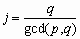.

Since k is the number of times the ant walked across the full width of the panel in the horizontal direction, it also gives the number of times the ant turned around at the left side of the panel. This number did not depend upon which strand the ant started, so every strand must meet the left side the same number of times. Since the total number of times all strands meet the left side of the panel is p, the number of different strands is, as desired (we could make the same argument using q and j and the top of the panel).

A nice consequence of this argument is that, since each component of the design meets the left (and right) side of the paneltimes and the top (and bottom) of the paneltimes: Each component is, on its own, a k ´j knotwork panel.

While this approach to the problem is very natural, it is difficult to extend it to other designs. We will describe another approach, based on the mathematics of permutations, which extends very nicely to several other designs. To begin with, imagine a vertical strip of a panel. A p ´ q panel has 2p strings running horizontally across it, intertwining with each other (several, or all, of these strings may belong to the same component). Starting at the left side, number the strings 1 to 2p from top to bottom (so strings 1 and 2 will be parts of the same component, as will 3 and 4, and so forth). As we follow the strings to the right, their positions change. We will look at how these positions change as we move to the right across one column of the original square grid; we denote this permutation sp. Figure 4 shows an example with p = 4.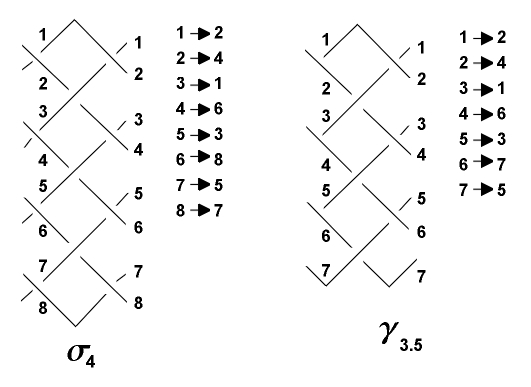Figure 4: Permutations of strings.

Permutations are often represented using cycle notation. For example, the permutation of the set {1, 2, 3} that sends 1 to 2, 2 to 3 and 3 to 1 is represented by the 3-cycle (123). The permutation of the set {1, 2, 3, 4, 5} which sends 1 to 2 and 2 to 1, 3 to 5 and 5 to 3 and leaves 4 fixed would be written (12)(35)(4). We can visualize these cycles as shown in Figure 5.Figure 5: Cycles in permutations

We can "multiply" permutations by performing one after the other and looking at the result. Products of permutations are traditionally read from right to left. For example, the product (123)(12)(35)(4) of the permutations in Figure 5 sends 1 to 2, and then 2 to 3, so the product sends 1 to 3. Similarly, we can see that the product sends 3 to 5, and 5 to 1, so we have a cycle (135). Finally, the product fixes 2 and 4. In cycle notation, we write that (123)(12)(35)(4) = (135)(2)(4).

Returning to our knotwork panel: In cycle notation, the permutation s4 in Figure 4 is (12468753). In general, the permutation is sp = (124...2p 2p-1 2p-3...53), which is always a 2p-cycle. When the strings hit the left or right sides of the panel, they "bounce" with a permutation dp = (12)(34)(56)...(2p-1 2p) because neighboring strings switch places.

So if we follow the strings from the left side of the panel to the right and back again, bouncing off each end, when we return to our original position (moving once again to the right), the total permutation is given by the product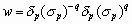. We want to simplify this permutation and split it into disjoint cycles (so no number appears in more than one cycle). At this point, each cycle will correspond to a component of the panel. In fact, each component of the design corresponds to two of these cycles: If we start at any point on the component, one cycle describes our path around the component moving in one direction, and the other cycle describes the path if we move in the other direction.

First note that(this is easy to check and is left as an exercise). So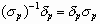, which means that we can rewrite w as(since dp has order 2). Now we use a fact about permutations: if we multiply a single cycle of length n by itself m times, the product will consist of gcd(n, m) disjoint cycles. Since sp is a single 2p-cycle, the number of disjoint cycles in w is gcd(2p, 2q) = 2gcd(p, q). Since each component of the panel corresponds to two of these cycles (traversing the component in both directions), the number of components is once again gcd(p, q).

In the following sections, we will extend this approach to several other basic designs.

2.2 Circular Borders. Circular motifs are also common in Celtic knotwork designs, often appearing as elements in larger designs, such as stone carvings or illuminated manuscripts [2, 8]. They are also found in modern designs influenced by the Celtic tradition.Figure 6: The 2.5 ´ 12 circular border has 1 component and the 2 ´ 12 circular border has
4 components. The 1.5 ´3 circular border (the Borromean link) has three components.

The simplest circular knotwork design is made by bending a p ´ q knotwork panel into a circle, and joining the left and right sides to get a p ´q circular border. Three such designs are shown in Figure 6. Cromwell  noted that knotwork panels must have an even number of horizontal strings in order to close up (a p ´q panel has 2p strings). In circular designs, however, there can be an odd number of strings, so p can be a half-integer as well as an integer. Figure 6 shows examples of each type. There is, once again, a simple formula for the number of components in the design. The simplest circular borders are well known to knot theorists, although by other names  the 1 ´ 3 circular border is the trefoil knot, and the 1.5 ´ 3 circular border is the Borromean link. Figure 6 shows the Borromean link; a tiny trefoil knot can be seen in the center of the design.

Theorem 2: The number of components in a p´ q circular border is gcd(2p, q).

Notice that when p is a half-integer, 2p is odd, so the design will have an odd number of components. As an example, in Figure 6, the figure on the left has gcd(5, 12) = 1 component, the figure in the middle has gcd(4, 12) = 4 components, and the Borromean link has gcd(3, 3) = 3 components. We will prove Theorem 2 using the permutation technique we developed in Section 2.1. We first consider the case when p is an integer, so the permutation across one "diamond" is once again sp. In the circular case, the permutation of the strings as we travel once around the design is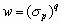. So, w splits into gcd(2p, q) disjoint cycles, each of which corresponds to a different component of the design. Unlike the case in Section 2.1, we are only following the strings in one direction, so we do not need to divide by 2. So the number of components is gcd(2p, q).

On the other hand, if p = k + 1/2 for some integer k, then we need to consider a slightly different permutation. In this case, there are 2p = 2k + 1 strings winding around the design. The permutation of the strings as we move one unit clockwise around the design (i.e. one "qth" of the way around) is given by gp = (1 2 4...2p-1 2p 2p-2...5 3), a 2p-cycle. The right illustration in Figure 4 shows the case when p = 3.5. So the permutation as we make a complete circuit of the design is. Since gp is a 2p-cycle, the number of disjoint cycles in w is once again gcd(2p,q), as desired.

2.3 Rectangular Borders. Our methods are easily adapted to looking at rectangular borders, or frames of constant width. In general, frames have three parameters: the height p, the breadth q and the width (of the band) n. We will refer to such a frame as a p ´ q frame of width n. Note that n, as for circles, may be either an integer or a half-integer, such as 1.5. Figure 7 shows several examples.Figure 7: Examples of frames: 5 by 5 of width 2, 5 by 5 of width 1.5, 5 by 6 of width 2, and 5 by 7 of width 2

The number of components in a frame design is given by the following theorem:

Theorem 3: The number of components in a p´q frame of width n is equal to 2gcd(|p q|, n) (if n is an integer) or to gcd(|p q|, 2n) (if n is a half-integer k + 1/2, with k an integer). Here we require 2n < min (p, q).

Notice that, as with circles, if the width of the frame is an integer then there is an even number of components, and if the width is a half-integer then there is an odd number of components. If we apply this result to the examples in Figure 7, we see that the designs (from left to right) have 2gcd(5  5, 2) = 4 strands (0 is divisible by anything), gcd(5  5, 3) = 3 strands, 2gcd(6  5, 2) = 2 strands and 2gcd(7  5, 2) = 4 strands. Once again, we will use permutations  as with the circular borders, the arguments are simpler and more natural than with the panels, since we do not need to worry about "bouncing" off the sides. The permutation of the strings of the design as we go along the sides is given by sn or gn, depending on whether n is an integer or a half-integer. In addition, we need to determine what happens as we "turn a corner" in the design. We will denote the permutation of the strings around a corner by an if n is an integer, and by bn if n is a half-integer. Figure 8 illustrates a4 and b3.5.

In general, we can see that an = (12)(34)(56)...(2n-1 2n); in fact, an is the same as dn from Section 2.1. Similarly, bn = (12)(34)(56)...(2n-2 2n-1)(2n) (note that in this case 2n is odd). Recall from Section 2.1 that. It is also easy to show that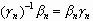.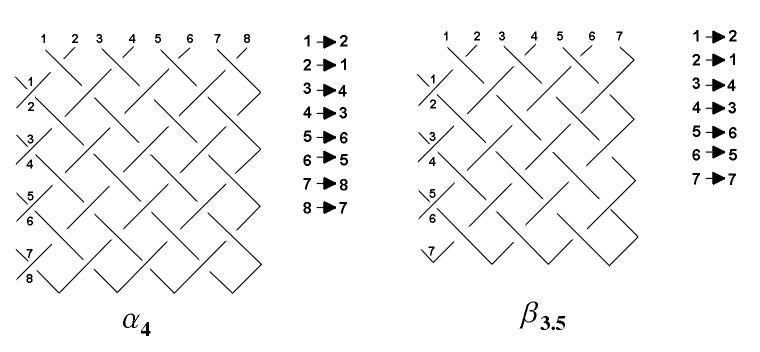Figure 8: Permutation of strings around corners.

If n is an integer, the permutation of the strings around the frame is given by the product of the four sides and four corners:The number of disjoint cycles in this permutation (and hence the number of strands in the design) is therefore gcd(|2(q  p)|, 2n) = 2gcd(|p  q|, n).

If n = k + 1/2, the permutation of the strings around the frame is given byThe number of disjoint cycles is therefore gcd(|2(q  p)|, 2n) = gcd(2|q  p|, 2k + 1). Since 2k + 1 is odd, the greatest common divisor must be odd, so we can ignore the factor of 2 in the first term. So the number of strands of the design is equal to gcd(|p  q|, 2n).

2.4 Half-frames or L shapes. We can apply the techniques we have developed to a wide range of designs involving a strip of constant width that makes right angle turns. A simple example of such a design is half of a frame, or an L shape. A p ´qL of width n has height p, base length q and is made from a strip of width n. As with panels, n must be an integer. Examples of L shapes are shown in Figure 9.

Theorem 4: The number of components (strands) in a p ´qL shape of width n is gcd(|p q|, n).Figure 9: Examples of L shapes:4 by 5 of width 2, 5 by 5 of width 2, 6 by 6 of width 2.

We can check this formula on the examples in Figure 9. The design on the left has gcd(5  4, 2) = 1 strand, the design in the middle has gcd(5  5, 2) = 2 strands, and the design on the right has gcd(6  6, 2) = 2 strands. We prove the formula using the same methods as before. Using the permutations we have defined in the earlier sections, the permutation of the strings in the L is obtained by beginning at the top, traveling to the bottom, turning a corner, traveling to the end, "bouncing" and eventually returning to our original position (after turning the corner and bouncing off the top to face down again). This permutation is. Using the relations we developed in the earlier sections, we can reduce this to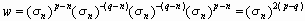.

So the number of disjoint cycles is gcd(2|p  q|, 2n) = 2gcd(|p  q|, n). As with knotwork panels, each component of the design is counted by two of these cycles, so the number of components is gcd(|p-q|, n).

3. Conclusion

Obviously, there is much more work to be done. Another common design is the Celtic Cross, and many other designs do not involve strips of constant width, but have strips of different widths joining with each other  these will require new techniques to analyze. And, of course, we have not even begun to analyze the effects of "breaking" the basic plaitwork pattern. We have presented a few basic ideas and results, but these are only a first step.

References

 Allen, J.R., Celtic Art in Pagan and Christian Times, Methuen, London, 1904

 Bain, G., Celtic Art: The Method of Construction, Dover, New York, 1973

 Cromwell, P.R., "Celtic Knotwork: Mathematical Art", Mathematical Intelligencer, Vol. 15, No. 1, 1993, pp. 36-47

 Doran, B., "Mathematical Sophistication of the Insular Celts  Spirals, Symmetries, and Knots as a Window onto their World View", Proceedings of the Harvard Celtic Colloquium, Vol. 15, 1995, pp. 258-289

 Gillman, P., http://www.boulder.net/~gillman/prism/drawing.html

 Grunbaum, B. and Shephard, G.C., "Interlace Patterns in Islamic and Moorish Art", Leonardo,, Vol. 25, No. 3/4, 1992, pp. 331-339

 Henry, F., Irish Art in the Early Christian Period, Cornell University Press, Ithaca N.Y., 1965

 Laing, L. and J., Art of the Celts, Thames and Hudson, London, 1992

 Meehan, A., Celtic Design: Knotwork, Thames and Hudson, New York, 1991

 Trilling, J., "Medieval Interlace Ornament: the Making of a Cross-Cultural Idiom", Arte Medievale, Vol. 9, 1995, pp. 59-86